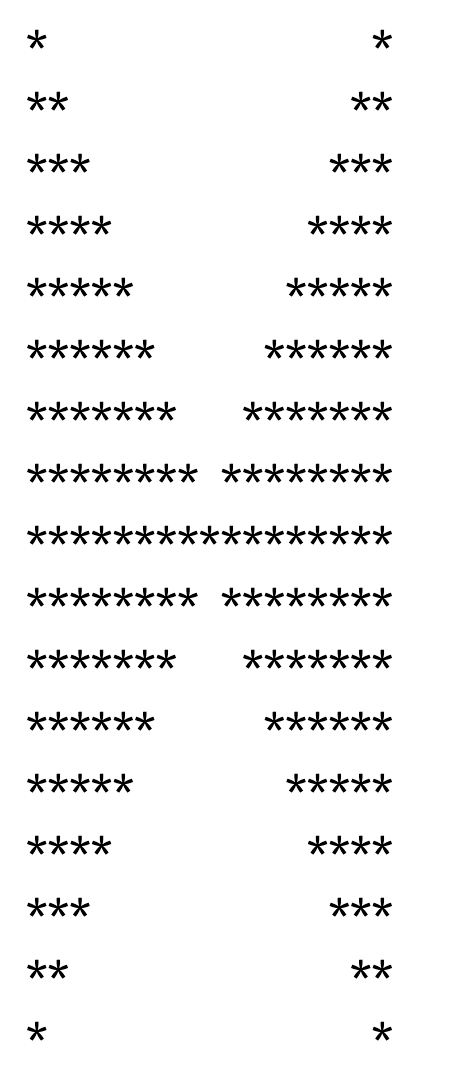# Code to draw a Big H with all stars

Coding is everything! Code Now!

# Background & symptoms

You like letter `H` ? (?? why I like H??). Anyway, for whatever reasons (to show off, for exam, code test, etc), you need to draw a big H, or two triangles which point to each other. Here is the code for your quick reference:

## Output preview## Designing planing

For `pattern` code test, here are some golden tips

• Normally you’d use two pointers, one for `row` another for `columns` , then compare their relationship to determine whether to output or not
• `TDD` is highly recommended to test boundary case and edge case

## Full source code

``````

import java.util.Arrays;
import java.util.Scanner;

public class Pattern {

public static void main(String[] args) {
System.out.println("please input a character for display");
Scanner scanner = new Scanner(System.in);
String pattern = scanner.nextLine();
if (pattern == null || pattern.isEmpty()) {
System.out.println("Invalid input pattern");
System.exit(1);
}
char ptn = pattern.trim().charAt(0);

System.out.println("please input levels (number smaller than 100)");
int level = scanner.nextInt();
if ( level > 100) {
System.out.println("Invalid input for level");
System.exit(1);
}
char[][] chars = drawPattern(ptn, level);
print2DArrays(chars);
}

private static void print2DArrays(char[][] chars) {
int row = chars.length;
for (int i = 0; i < row; i++) {
int col = chars[i].length;
for (int j = 0; j < col; j++) {
System.out.print(chars[i][j]);
}
System.out.println();
}
}

private static char[][] drawPattern(char ptn, int level) {
int len = 2 * level;
int oppNum = 2 * level - 2;
char[][] pattern = new char[len - 1][len - 1];

for (int i = 0; i < len - 1; i++) {
for (int j = 0; j < len - 1; j++) {
pattern[i][j] = ' ';
if (i < level) {
if (j <= i || j >= (oppNum - i)) {
pattern[i][j] = ptn;
}
} else {
if (j <= (oppNum - i) || j >= i) {
pattern[i][j] = ptn;
}
}

}

}
return pattern;
}
}

``````

# Code explanation!

Above code is quite self-explanation. However here are some quick note-worthy points:

• You can enter whatever patterns you want to form this big H (not only star)
• Essentially this is using two pointers representing row anc column, then compare their relationship to determine whether to print out or not.

–End–

Updated: